1. 所有题目来源为力扣
2. 题解为原创，仅为个人当前阶段能想到/能接受的解法，不一定是优解
3. 🟢简单 🟠中等 🔴困难 ⚪未完成 ⚫未更新

4. 有回顾价值题集：

5. 其它题解：我的 LeetCode 题解

# 🟢 2021.11.01 (237. 删除链表中的节点)

## 题目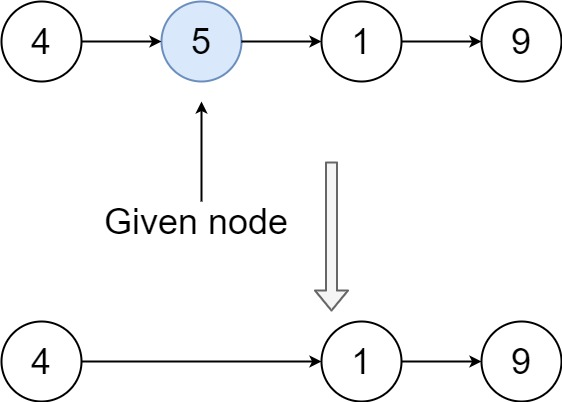• 链表中节点的数目范围是 [2, 1000]
• -1000 <= Node.val <= 1000
• 链表中每个节点的值都是唯一的
• 需要删除的节点 node链表中的一个有效节点 ，且 不是末尾节点

## 题解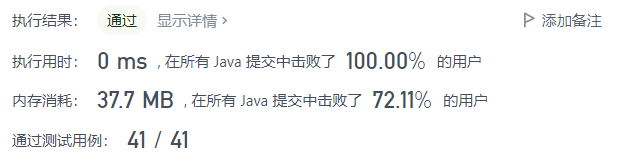# 🟢 2021.10.31 (500. 键盘行)

## 题目

• 第一行由字符 "qwertyuiop" 组成。
• 第二行由字符 "asdfghjkl" 组成。
• 第三行由字符 "zxcvbnm" 组成。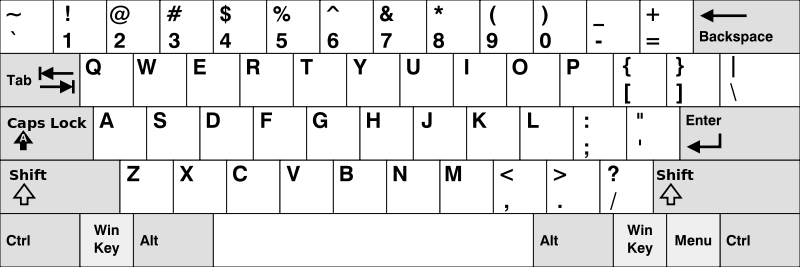• 1 <= words.length <= 20
• 1 <= words[i].length <= 100
• words[i] 由英文字母（小写和大写字母）组成

## 题解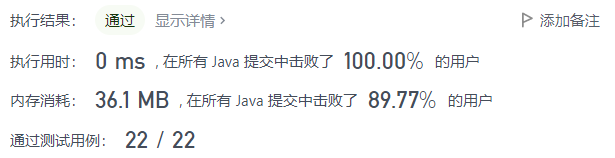# 🟠 2021.10.30 (260. 只出现一次的数字 III)

## 题目

• 2 <= nums.length <= 3 * 10^4
• -2^31 <= nums[i] <= 2^31 - 1
• 除两个只出现一次的整数外，nums 中的其他数字都出现两次

## 题解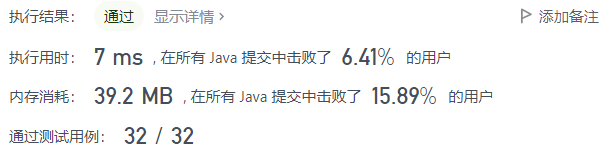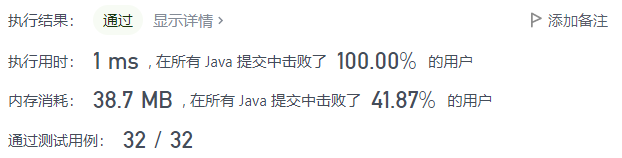🔴 未做

# 🟠 2021.10.28 (869. 重新排序得到 2 的幂)

## 题目

1. 1 <= N <= 10^9

## 题解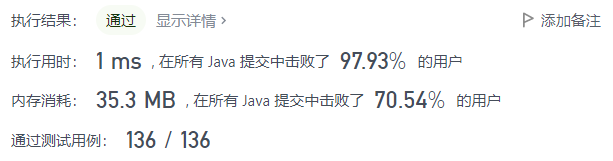# 🔴 2021.10.27 (301. 删除无效的括号)

## 题目

• 1 <= s.length <= 25
• s 由小写英文字母以及括号 '('')' 组成
• s 中至多含 20 个括号

## 题解

• 若扫描并加入了一个左括号，则 bias++
• 若扫描并加入了一个右括号，则 bias--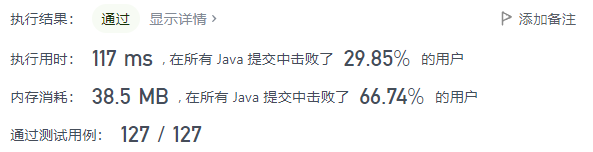# 🟢 2021.10.26 (496. 下一个更大元素 I)

## 题目

nums1 中数字 x 的下一个更大元素是指 xnums2 中对应位置的右边的第一个比 x 大的元素。如果不存在，对应位置输出 -1

• 1 <= nums1.length <= nums2.length <= 1000
• 0 <= nums1[i], nums2[i] <= 10^4
• nums1nums2中所有整数 互不相同
• nums1 中的所有整数同样出现在 nums2

## 题解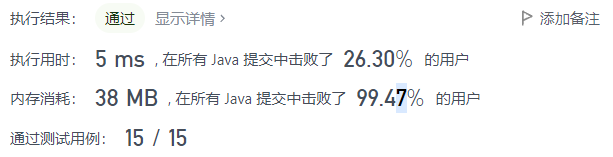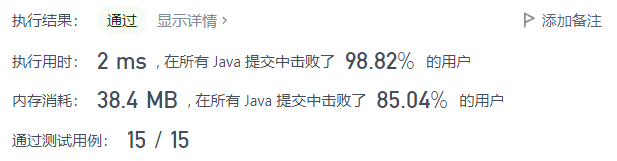# 🟠 2021.10.25 (240. 搜索二维矩阵 II)

## 题目

• 每行的元素从左到右升序排列。
• 每列的元素从上到下升序排列。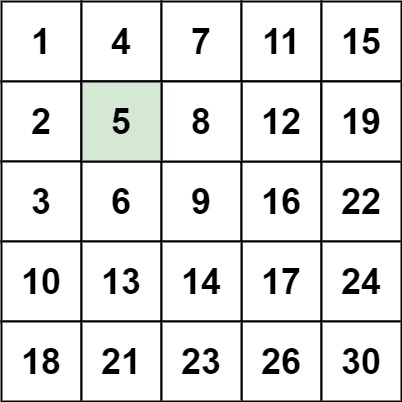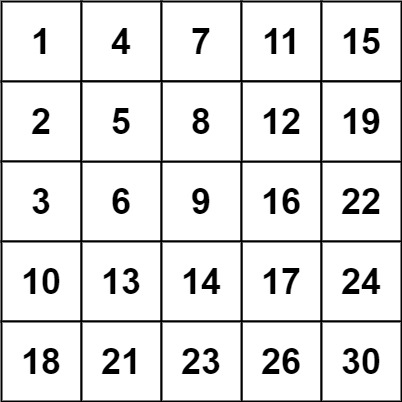• m == matrix.length
• n == matrix[i].length
• 1 <= n, m <= 300
• -10^9 <= matrix[i][j] <= 10^9
• 每行的所有元素从左到右升序排列
• 每列的所有元素从上到下升序排列
• -10^9 <= target <= 10^9

## 题解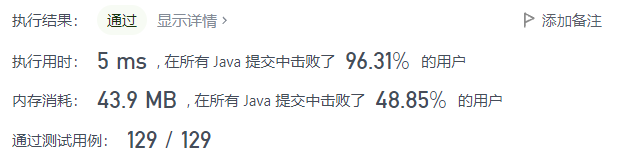🟠 未解决

# 🟢 2021.10.23 (492. 构造矩形)

## 题目

1. 给定的面积不大于 10,000,000 且为正整数。
2. 你设计的页面的长度和宽度必须都是正整数。

## 题解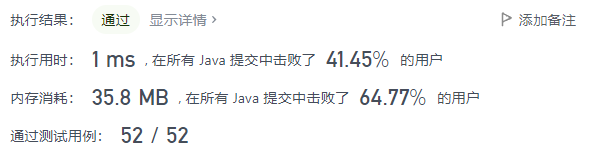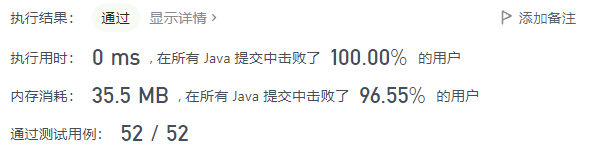# 🟠 2021.10.22 (229. 求众数 II)

## 题目

• 1 <= nums.length <= 5 * 10^4
• -10^9 <= nums[i] <= 10^9

## 题解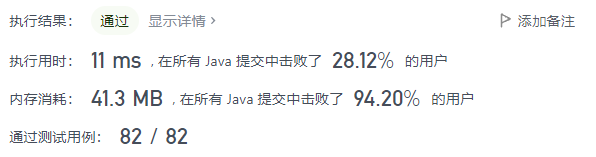# 🟢 2021.10.21 (66. 加一)

## 题目

• 1 <= digits.length <= 100
• 0 <= digits[i] <= 9

## 题解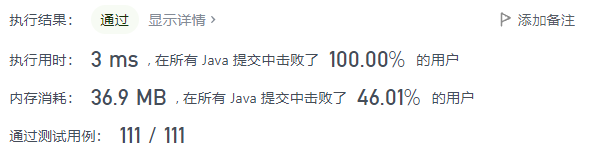🟢 未做

# 🟠 2021.10.19 (211. 添加与搜索单词)

## 题目

• WordDictionary() 初始化词典对象
• void addWord(word)word 添加到数据结构中，之后可以对它进行匹配
• bool search(word) 如果数据结构中存在字符串与 word 匹配，则返回 true ；否则，返回 false 。word 中可能包含一些 ‘.’ ，每个 . 都可以表示任何一个字母。

• 1 <= word.length <= 500
• addWord 中的 word 由小写英文字母组成
• search 中的 word 由 ‘.’ 或小写英文字母组成
• 最多调用 50000addWordsearch

## 题解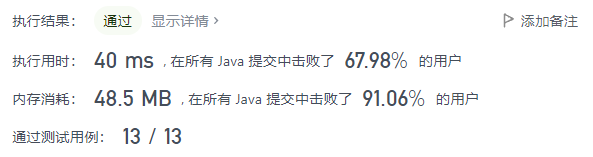# 🟢 2021.10.18 (476. 数字的补数)

## 题解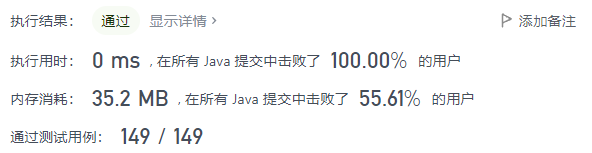🔴 事务繁忙，无空做

🔴 事务繁忙，无空做

# 🟠 2021.10.15 (38. 外观数列)

## 题目

「外观数列」是一个整数序列，从数字 1 开始，序列中的每一项都是对前一项的描述。

• countAndSay(1) = "1"
• countAndSay(n) 是对 countAndSay(n-1) 的描述，然后转换成另一个数字字符串。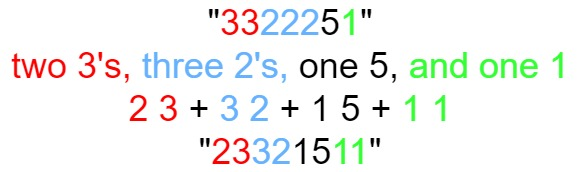• 1 <= n <= 30

## 题解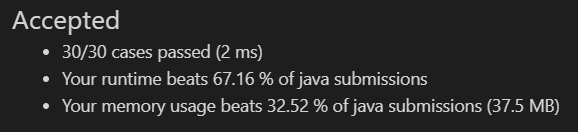# 🟢 2021.10.14 (剑指 Offer II 069. 山峰数组的顶部)

## 题解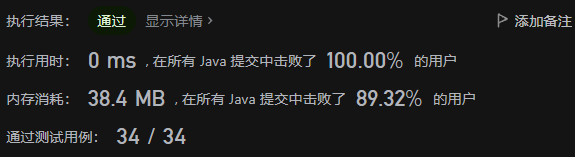# 🟢 2021.10.13 (412. Fizz Buzz)

## 题目

\1. 如果 n 是3的倍数，输出“Fizz”；

\2. 如果 n 是5的倍数，输出“Buzz”；

\3. 如果 n 同时是3和5的倍数，输出 “FizzBuzz”。

## 题解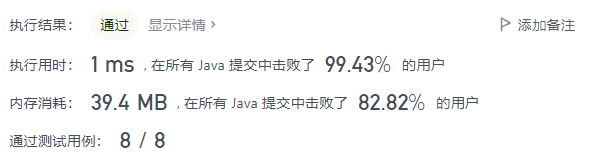# 🟠 2021.10.12 (29. 两数相除)

## 题目

• 被除数和除数均为 32 位有符号整数。
• 除数不为 0。
• 假设我们的环境只能存储 32 位有符号整数，其数值范围是 [−2^31, 2^31 − 1]。本题中，如果除法结果溢出，则返回 2^31 − 1。除数不为 0。

## 题解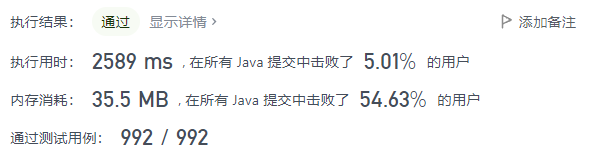# 🔴 2021.10.11 (273. 整数转换英文表示)

## 题目

• 0 <= num <= 231 - 1

## 题解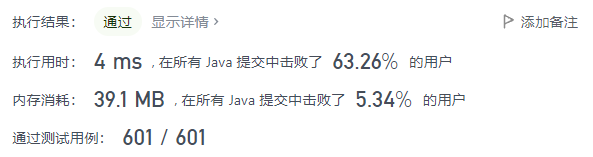# 🟢 2021.10.10 (441. 排列硬币)

## 题目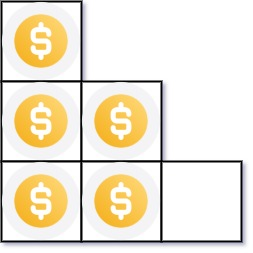• 1 <= n <= 2^31 - 1

## 题解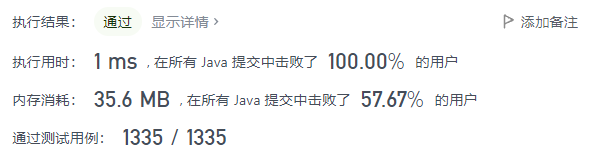🔴 不会，也没看题解，没空

# 🟠 2021.10.08 (187. 重复的DNA序列)

## 题目

• 0 <= s.length <= 105
• s[i]'A''C''G''T'

## 题解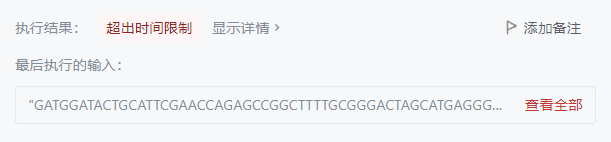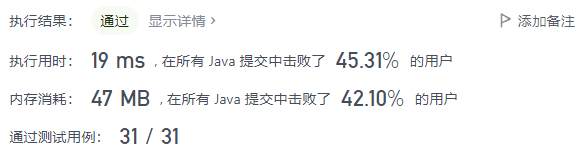# 🟢 2021.10.07 (434. 字符串中的单词数)

## 题解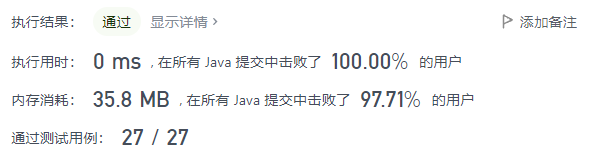# 🟢 2021.10.06 (414. 第三大的数)

## 题目

• 1 <= nums.length <= 10^4
• -2^31 <= nums[i] <= 2^31 - 1

## 题解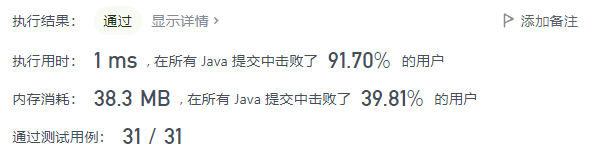# 🟠 2021.10.05 (284. 窥探迭代器)

## 题目

• PeekingIterator(int[] nums) 使用指定整数数组 nums 初始化迭代器。
• int next() 返回数组中的下一个元素，并将指针移动到下个元素处。
• bool hasNext() 如果数组中存在下一个元素，返回 true ；否则，返回 false
• int peek() 返回数组中的下一个元素，但 移动指针。

## 题解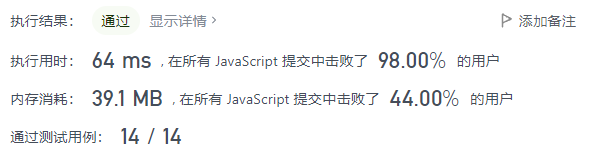# 🟢 2021.10.04 (482. 密钥格式化)

## 题目

1. S 的长度可能很长，请按需分配大小。K 为正整数。
2. S 只包含字母数字（a-z，A-Z，0-9）以及破折号’-‘
3. S 非空

## 题解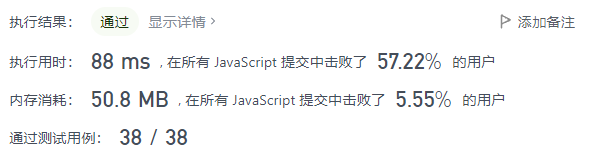# 🟠 2021.10.03 (166. 分数到小数)

## 题目

• -2^31 <= numerator, denominator <= 2^31 - 1
• denominator != 0

## 题解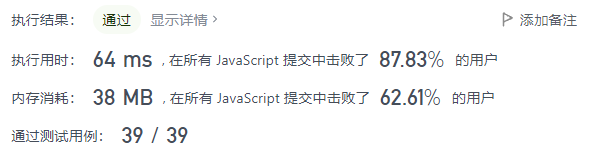# 🟢 2021.10.02 (405. 数字转换为十六进制数)

## 题目

1. 十六进制中所有字母(a-f)都必须是小写。
2. 十六进制字符串中不能包含多余的前导零。如果要转化的数为0，那么以单个字符'0'来表示；对于其他情况，六进制字符串中的第一个字符将不会是0字符。
3. 给定的数确保在32位有符号整数范围内。
4. 不能使用任何由库提供的将数字直接转换或格式化为十六进制的方法。

## 题解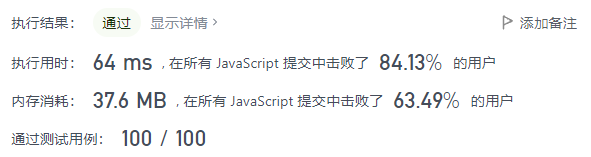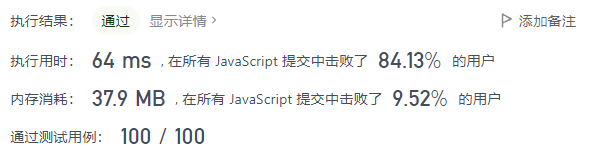# 🟢 2021.10.01 (1436. 旅行终点站)

## 题目

• 1 <= paths.length <= 100
• paths[i].length == 2
• 1 <= cityAi.length, cityBi.length <= 10
• cityAi != cityBi
• 所有字符串均由大小写英文字母和空格字符组成。

## 题解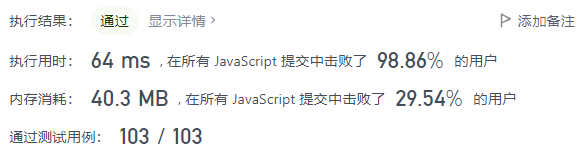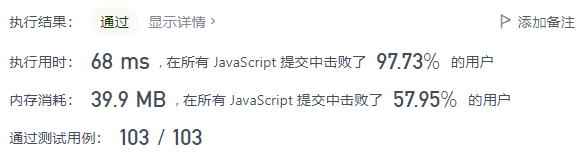# 🟠 2021.09.30 (223. 矩形面积)

## 题目

• 第一个矩形由其左下顶点 (ax1, ay1) 和右上顶点 (ax2, ay2) 定义。
• 第二个矩形由其左下顶点 (bx1, by1) 和右上顶点 (bx2, by2) 定义。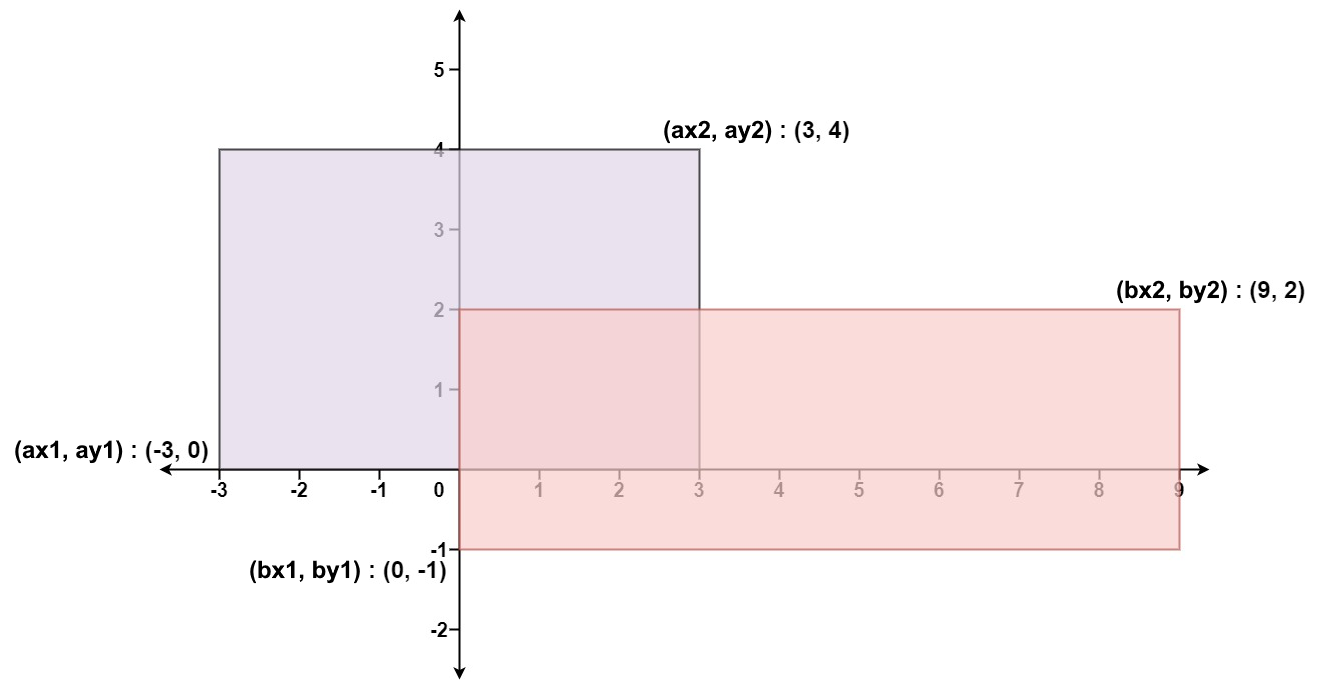• -104 <= ax1, ay1, ax2, ay2, bx1, by1, bx2, by2 <= 104

## 题解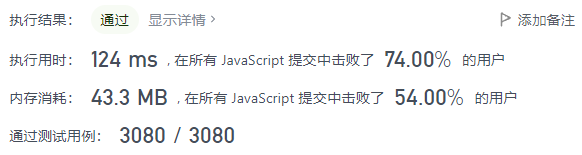# 🔴 2021.09.29 (517. 超级洗衣机)

## 题目

• n == machines.length
• 1 <= n <= 104
• 0 <= machines[i] <= 105

## 题解

$O(n^3)$ 暴力超时代码（困难题果然还是不能暴力的呢）：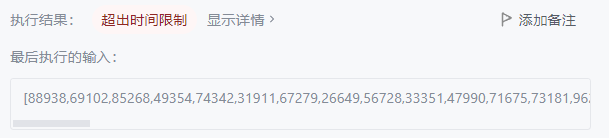$O(n)$ AC 完整代码：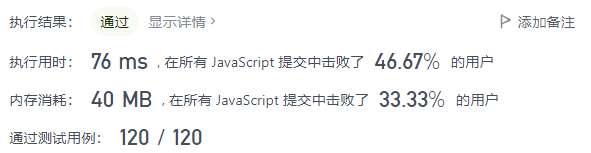# 🟠 2021.09.28 (437. 路径总和 III)

## 题目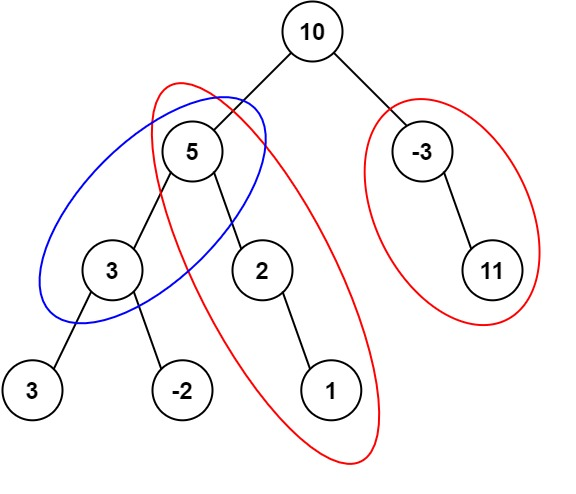## 题解# ⚪ 2021.09.27 (639. 解码方法 II)

🔴 速通 JavaScript 去了，未做

🟠 未更新

# 🟠 2021.09.25 (583. 两个字符串的删除操作)

## 题目

1. 给定单词的长度不超过500。
2. 给定单词中的字符只含有小写字母。

## 题解

• word1[i] == word2[j] 则显然 dp[i, j] = dp[i-1, j-1] + 1，表示将当前比较的位加入到前一次的最长公共子序列长度中；
• word1[i] == word2[j] ，则继承两种上一状态中长度较长的那个长度作为最长长度，即 dp[i, j] = Max(dp[i-1, j], dp[i, j-1])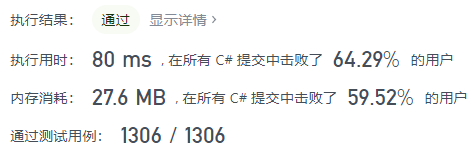# 🟠 2021.09.24 (430. 扁平化多级双向链表)

## 题目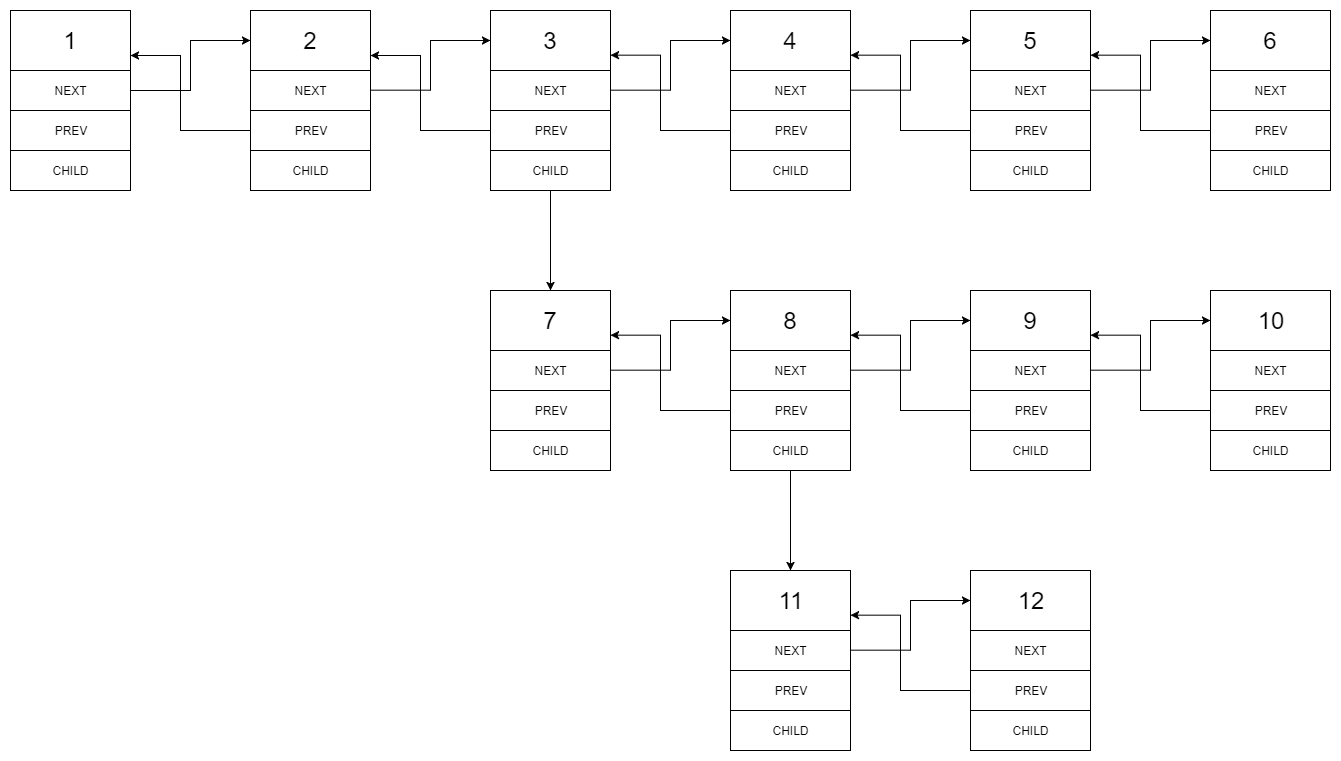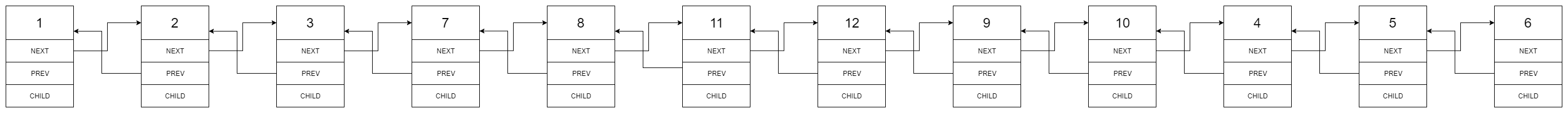1—-2—-NULL
|
3—-NULL

• 节点数目不超过 1000
• 1 <= Node.val <= 10^5

## 题解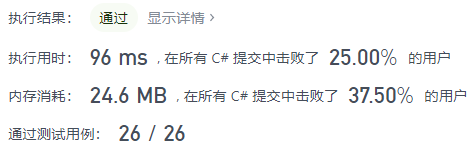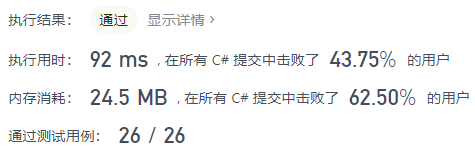# 🟢 2021.09.23 (326. 3的幂)

## 题解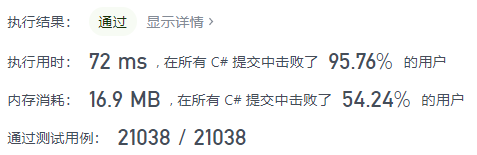# 🟠 2021.09.22 (725. 分隔链表)

## 题目

k 个部分应该按照在链表中出现的顺序排列，并且排在前面的部分的长度应该大于或等于排在后面的长度。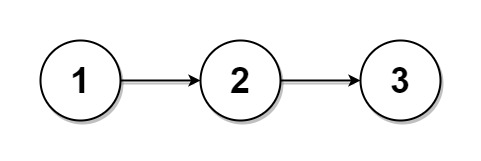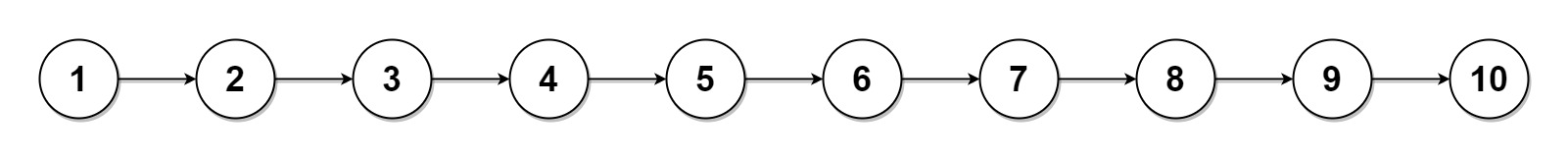• 链表中节点的数目在范围 [0, 1000]
• 0 <= Node.val <= 1000
• 1 <= k <= 50

## 题解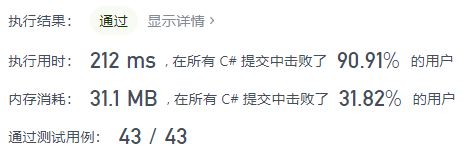# 🟢 2021.09.21 (58. 最后一个单词的长度)

## 题目

• 1 <= s.length <= 104
• s 仅有英文字母和空格 ' ' 组成
• s 中至少存在一个单词

## 题解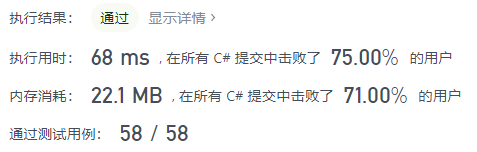# 🟠 2021.09.20 (673. 最长递增子序列的个数)

## 题解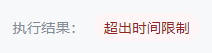Update：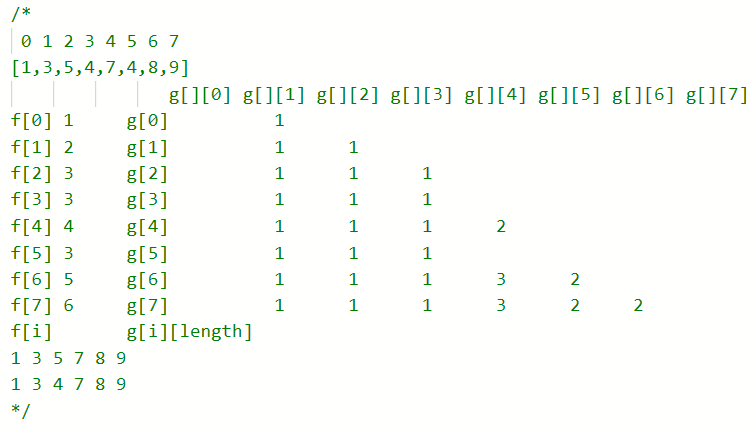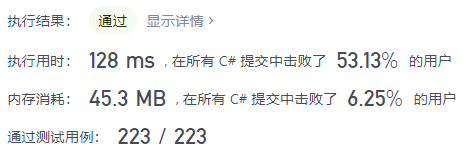# 🟠 2021.09.19 (650. 只有两个键的键盘)

## 题目

• Copy All（复制全部）：复制这个记事本中的所有字符（不允许仅复制部分字符）。
• Paste（粘贴）：粘贴 上一次 复制的字符。

• 1 <= n <= 1000

## 题解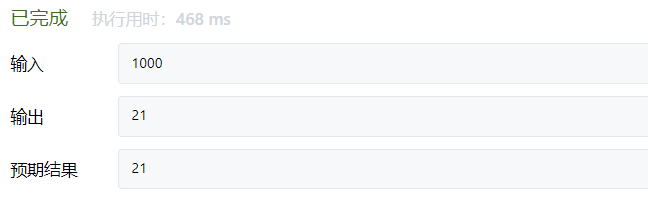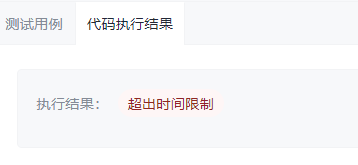How many characters may be there in the clipboard at the last step if n = 3? n = 7? n = 10? n = 24?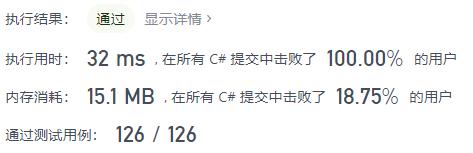# 🟢 2021.09.18 (292. Nim 游戏)

## 题目

• 桌子上有一堆石头。
• 你们轮流进行自己的回合，你作为先手。
• 每一回合，轮到的人拿掉 1 - 3 块石头。
• 拿掉最后一块石头的人就是获胜者。

## 题解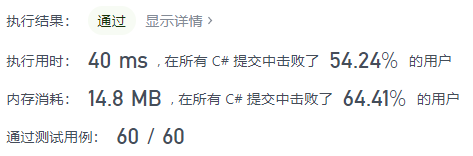# 🟠 2021.09.17 (36. 有效的数独)

## 题目

1. 数字 1-9 在每一行只能出现一次。
2. 数字 1-9 在每一列只能出现一次。
3. 数字 1-9 在每一个以粗实线分隔的 3x3 宫内只能出现一次。（请参考示例图）

• 一个有效的数独（部分已被填充）不一定是可解的。
• 只需要根据以上规则，验证已经填入的数字是否有效即可。

[[“5”,”3”,”.”,”.”,”7”,”.”,”.”,”.”,”.”]
,[“6”,”.”,”.”,”1”,”9”,”5”,”.”,”.”,”.”]
,[“.”,”9”,”8”,”.”,”.”,”.”,”.”,”6”,”.”]
,[“8”,”.”,”.”,”.”,”6”,”.”,”.”,”.”,”3”]
,[“4”,”.”,”.”,”8”,”.”,”3”,”.”,”.”,”1”]
,[“7”,”.”,”.”,”.”,”2”,”.”,”.”,”.”,”6”]
,[“.”,”6”,”.”,”.”,”.”,”.”,”2”,”8”,”.”]
,[“.”,”.”,”.”,”4”,”1”,”9”,”.”,”.”,”5”]
,[“.”,”.”,”.”,”.”,”8”,”.”,”.”,”7”,”9”]]

## 题解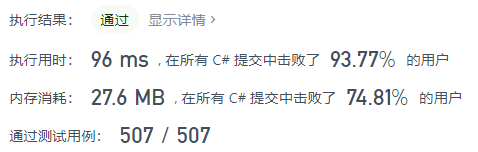# 🟠 2021.08.25 (797. 所有可能的路径)

## 题目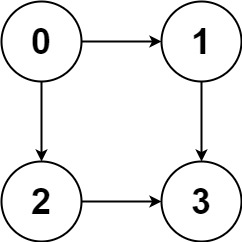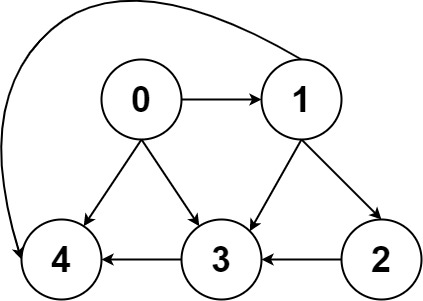• n == graph.length
• 2 <= n <= 15
• 0 <= graph[i][j] < n
• graph[i][j] != i（即，不存在自环）
• graph[i] 中的所有元素 互不相同
• 保证输入为 有向无环图（DAG）

## 题解（回溯搜索）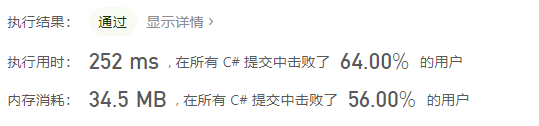# 🟠 2021.08.24 (787. K 站中转内最便宜的航班)

## 题目

n 个城市通过一些航班连接。给你一个数组 flights ，其中 flights[i] = [from_i, to_i, price_i] ，表示该航班都从城市 from_i 开始，以价格 price_i 抵达 to_i

n = 3, edges = [[0,1,100],[1,2,100],[0,2,500]]
src = 0, dst = 2, k = 1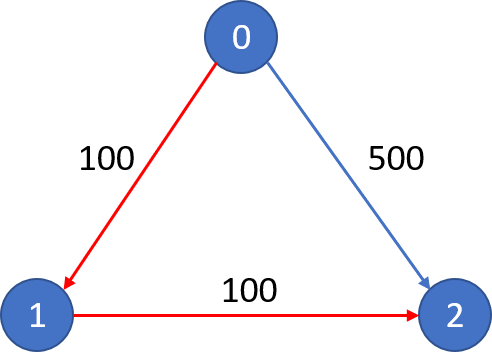n = 3, edges = [[0,1,100],[1,2,100],[0,2,500]]
src = 0, dst = 2, k = 0

• 1 <= n <= 100
• 0 <= flights.length <= (n * (n - 1) / 2)
• flights[i].length == 3
• 0 <= from_i, to_i < n
• from_i != to_i
• 1 <= price_i <= 104
• 航班没有重复，且不存在自环
• 0 <= src, dst, k < n
• src != dst

## 题解（BFS+剪枝）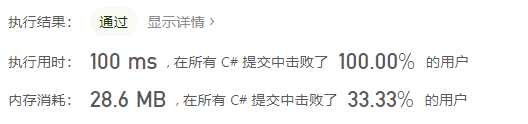# 🟢 2021.08.23 (1646. 获取生成数组中的最大值)

## 题目

• nums = 0
• nums = 1
• 2 <= 2 * i <= n 时，nums[2 * i] = nums[i]
• 2 <= 2 * i + 1 <= n 时，nums[2 * i + 1] = nums[i] + nums[i + 1]

• 0 <= n <= 100

## 题解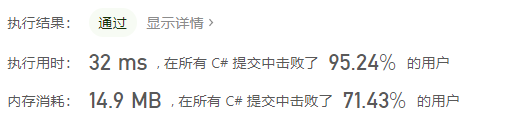# 🟠 2021.08.22 (789. 逃脱阻碍者)

## 题目

• 1 <= ghosts.length <= 100
• ghosts[i].length == 2
• -104 <= xi, yi <= 104
• 同一位置可能有 多个阻碍者
• target.length == 2
• -104 <= xtarget, ytarget <= 104

## 题解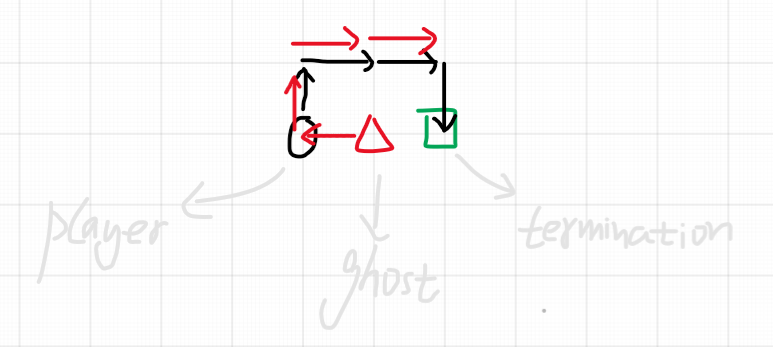Return true if it is possible to escape, otherwise return false.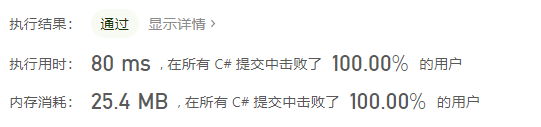# 🟠 2021.08.21 (443. 压缩字符串)

## 题目

• 如果这一组长度为 1 ，则将字符追加到 s 中。
• 否则，需要向 s 追加字符，后跟这一组的长度。

“aa” 被 “a2” 替代。”bb” 被 “b2” 替代。”ccc” 被 “c3” 替代。

• 1 <= chars.length <= 2000
• chars[i] 可以是小写英文字母、大写英文字母、数字或符号

## 题解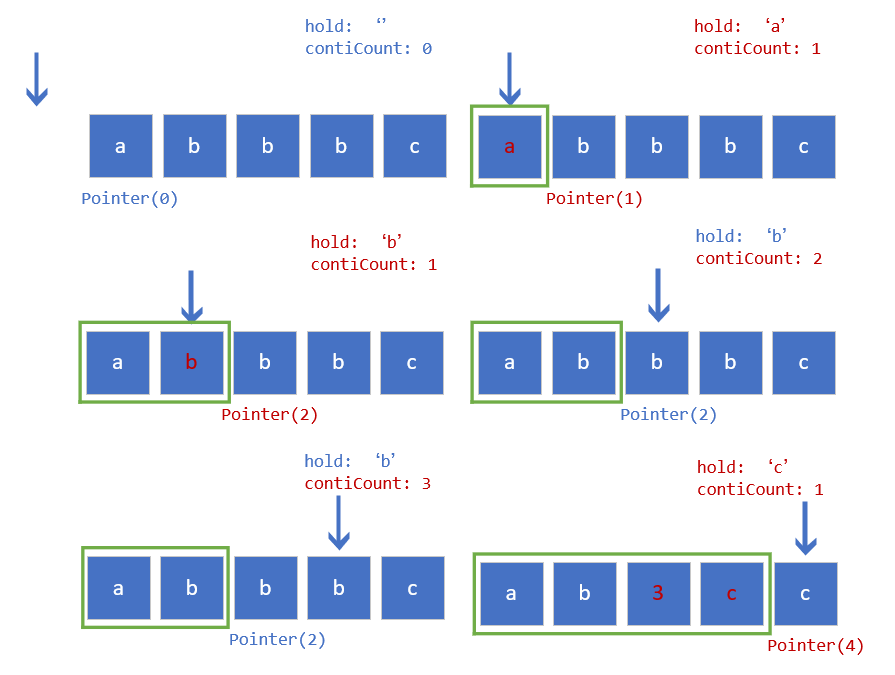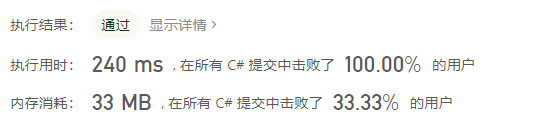# 🟢 2021.08.20 (541. 反转字符串 II)

## 题目

• 如果剩余字符少于 k 个，则将剩余字符全部反转。
• 如果剩余字符小于 2k 但大于或等于 k 个，则反转前 k 个字符，其余字符保持原样。

• 1 <= s.length <= 104
• s 仅由小写英文组成
• 1 <= k <= 104

## 题解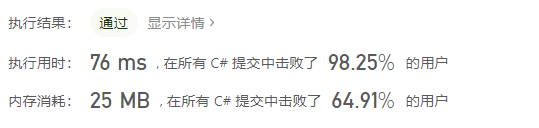# 🟢 2021.08.19 (345. 反转字符串中的元音字母)

## 题目

• 元音字母不包含字母 “y” 。

## 题解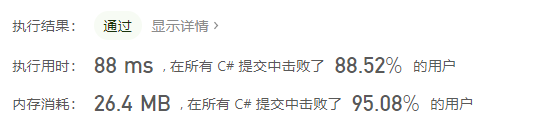# 🔴 2021.08.18 (552. 学生出勤记录 II)

## 题目

• 'A'：Absent，缺勤
• 'L'：Late，迟到
• 'P'：Present，到场

• 总出勤 计，学生缺勤（'A'严格 少于两天。
• 学生 不会 存在 连续 3 天或 连续 3 天以上的迟到（'L'）记录。

“PP” , “AP”, “PA”, “LP”, “PL”, “AL”, “LA”, “LL”

• 1 <= n <= 10^5

## 题解（DFS+记忆化搜索）

DFS+记忆化搜索版完整代码：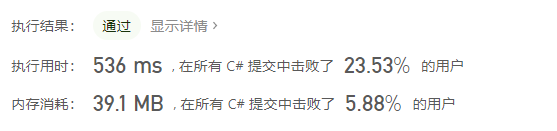# 🟢 2021.08.17 (551. 学生出勤记录 I)

## 题目

• 'A'：Absent，缺勤
• 'L'：Late，迟到
• 'P'：Present，到场

• 总出勤 计，学生缺勤（'A'严格 少于两天。
• 学生 不会 存在 连续 3 天或 连续 3 天以上的迟到（'L'）记录。

• 1 <= s.length <= 1000
• s[i]'A''L''P'

## 题解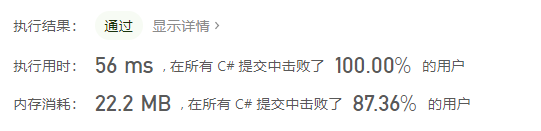# 🟠 2021.08.16 (526. 优美的排列)

## 题目

1. i 位的数字能被 i 整除
2. i 能被第 i 位上的数字整除

1. N 是一个正整数，并且不会超过15。

## 题解（DFS）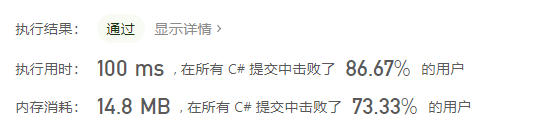# 🟠 2021.08.15 (576. 出界的路径数)

## 题目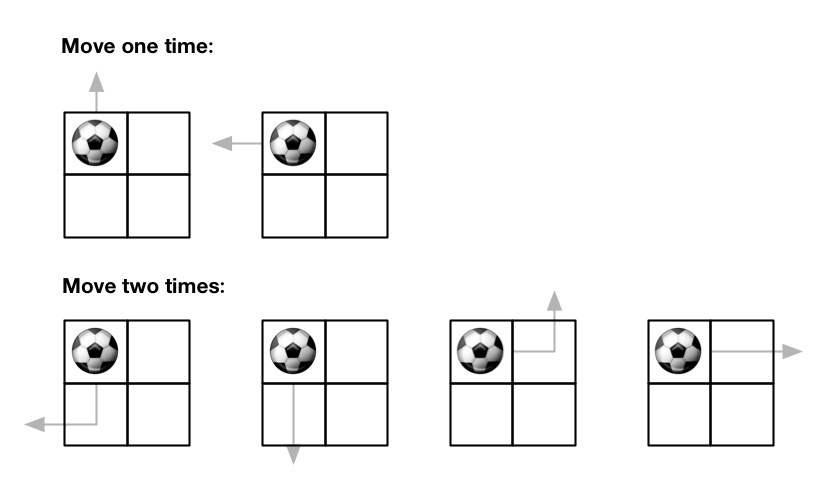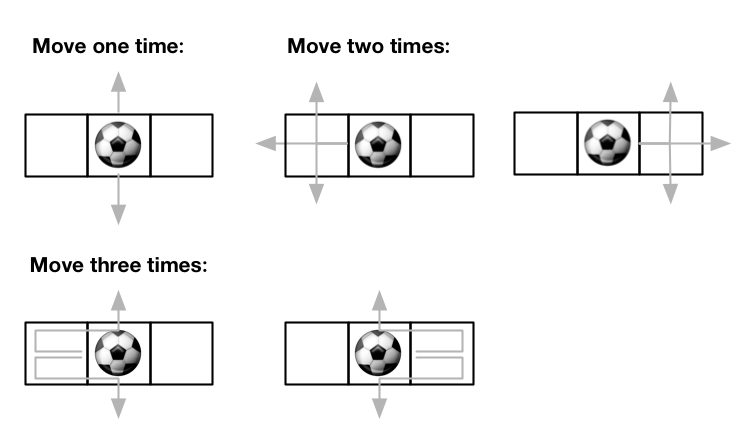• 1 <= m, n <= 50
• 0 <= maxMove <= 50
• 0 <= startRow < m
• 0 <= startColumn < n

## 题解（DFS+记忆化搜索）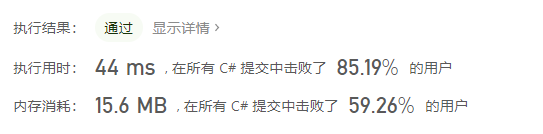🟠 未做

🔴 不会

# ⚪ 2021.08.12 (516. 最长回文子序列)

## 题目

• 1 <= s.length <= 1000
• s 仅由小写英文字母组成

🟠 待更新

🔴 不会

# 🟠 2021.08.10 (413. 等差数列划分)

## 题目

• 例如，[1,3,5,7,9][7,7,7,7][3,-1,-5,-9] 都是等差数列。

• 1 <= nums.length <= 5000
• -1000 <= nums[i] <= 1000

## 题解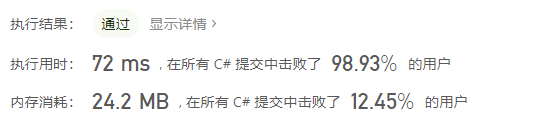# 🟠 2021.08.09 (313. 超级丑数)

## 题目

• 1 <= n <= 106
• 1 <= primes.length <= 100
• 2 <= primes[i] <= 1000
• 题目数据 保证 primes[i] 是一个质数
• primes 中的所有值都 互不相同 ，且按 递增顺序 排列

## 题解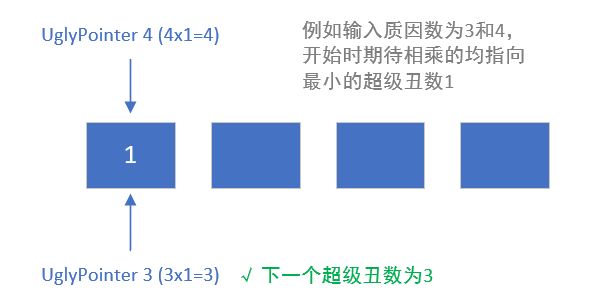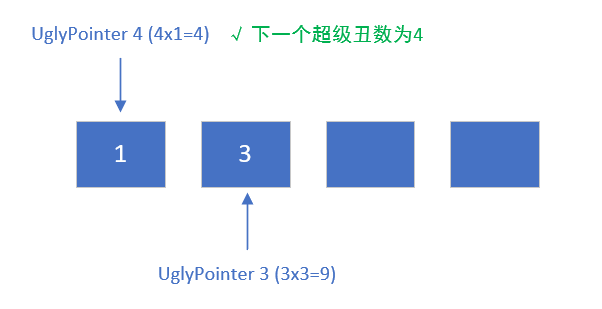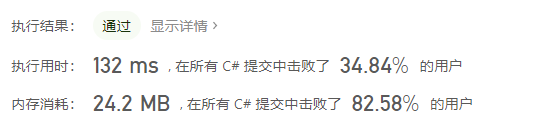0%